« Multistorey plane dual systems Space frames »

Comparison of multistorey frame and dual systems

The behaviour of the most common structural systems will be now examined. The structural system comprising only columns is called a frame system, while the one comprising both columns and walls is called a dual system.

The comparison of the two systems will be performed by means of examples. The structures under consideration are those used in the two previous paragraphs while the corresponding number of storeys is 4, 8 and 15. Two main quantities are compared, an actual one and an idealised one. The displacement of each level is the actual quantity and the equivalent column cross-section of each storey is the idealised one. The term equivalent column defines a theoretical column which develops a displacement equal to that developed by all storey columns. Infinite types of such idealised equivalent columns could be considered, however the fixed-ended one with 300 mm width is selected here. Alternatively, the rectangular fixed-ended column could be considered as equivalent.

In the comparison of the two systems, the distribution and not the magnitude of the seismic forces plays an important role. Consequently, the triangular distribution is used, approximating to the extent possible the actual distribution [*]NoteThe distribution of seismic forces derives from the modal response spectrum analysis method, described in chapter 6. of the majority of buildings designed by an engineer. In the related software the seismic forces are imposed on the considered building by selecting Apply Seismic Forces=ON in the dialog located in the central menu: "Horizontal Forces" à "Seismic Forces".

The three examples under consideration in both frame and dual versions are included in the related software. The modelling accounts for the elasticstiffnesses (Beam & Column Inertia Factor=1), the rigid bodies effect (Rigid Body=1) and the shear effect ( Shear Effect=1).

Stiffness parameter values and values for seismic forces are listed below.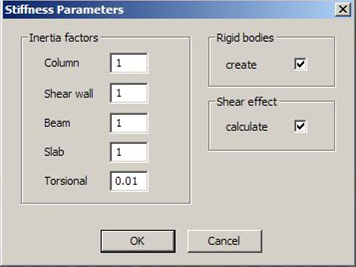Figure 5.3.3-1 Figure 5.3.3-1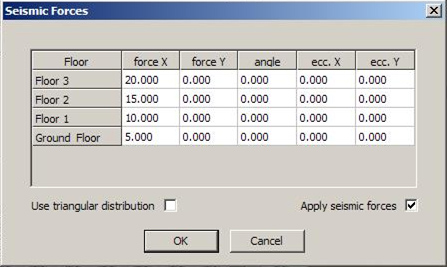Figure 5.3.3-2 Figure 5.3.3-2

The results derived from the software analysis are listed in the following tables and figures.

Frame Structural System (project <B_533a>)
Storey Force
H
Shear
force V
Displacement
δtotal
Displacement
δrelative
Σ(K) x 106 Equivalent
rectangular column
Equivalent
column with
b=300
kN kN mm mm N/m mm mm
0 5 50 0.801 0.801 62 483 569
1 10 45 1.766 0.965 47 449 517
2 15 35 2.532 0.766 46 447 513
3 20 20 2.983 0.451 44 442 505

Dual Structural System (project <B_533b>)
Storey Force
H
Shear
force V
Displacement
δtotal
Displacement
δrelative
Σ(K) x 106 Equivalent
rectangular column
Equivalent
column with
b=300
kN kN mm mm N/m mm mm
0 5 50 0.117 0.117 427 801 1169
1 10 45 0.341 0.224 201 655 872
2 15 35 0.580 0.239 146 603 775
3 20 20 0.792 0.212 94 537 660

Frame Structural System (project <B_533c>)
Storey Force H Shear
force V
Displacement
δtotal
Displacement δrelative Σ(K) x 106 Equivalent
rectangular column
Equivalent
column with
b=300
kN kN mm mm N/m mm mm
0 5 180 2.941 2.94 61 480 566
1 10 175 6.780 3.85 45 444 509
2 15 165 10.538 3.74 44 442 505
3 20 150 13.991 3.45 43 439 501
4 25 130 17.031 3.04 43 439 501
5 30 105 19.539 2.51 42 437 497
6 35 75 21.397 1.86 40 431 489
7 40 40 22.511 1.11 36 420 471

Dual Structural System (project <B_533d>)
Storey Force H Shear
force V
Displacement
δtotal
Displacement δrelative Σ(K) x 106 Equivalent
rectangular column
Equivalent
column with
b=300
kN kN mm mm N/m mm mm
0 5 180 0.488 0.49 367 769 1100
1 10 175 1.511 1.02 172 629 823
2 15 165 2.760 1.25 132 587 747
3 20 150 4.053 1.29 116 567 712
4 25 130 5.280 1.23 106 554 689
5 30 105 6.374 1.09 96 540 665
6 35 75 7.311 0.93 81 517 626
7 40 40 8.079 0.78 51 459 532

Frame Structural System (project <B_533e>)
Storey Force H Shear force V Displacement
δtotal
Displacement
δrelative
Σ(K) x 106 Equivalent
rectangular
column
Equivalent
column with
b=300
kN kN mm mm N/m mm mm
0 5 600 9.939 9.9 60 478 563
1 10 595 23.384 13.4 44 442 505
2 15 585 37.166 13.8 42 437 497
3 20 570 50.950 13.8 41 434 493
4 25 550 64.571 13.6 40 431 489
5 30 525 77.880 13.3 39 428 484
6 35 495 90.728 12.8 39 428 484
7 40 460 102.971 12.2 38 426 480
8 45 420 114.467 11.5 37 423 476
9 50 375 125.075 10.6 35 417 467
10 55 325 134.658 9.6 34 414 462
11 60 270 143.085 8.4 32 407 452
12 65 210 150.226 7.1 29 397 437
13 70 145 155.958 5.7 25 382 416
14 75 75 160.198 4.2 18 352 371
600 6200

Dual Structural System (project <B_533f>)
Storey Force H Shear force V Displacement
δtotal
Displacement
δrelative
Σ(K) x 106 Equivalent
rectangular
column
Equivalent
column with
b=300
kN kN mm mm N/m mm mm
0 5 600 1.797 1.8 333 749 1059
1 10 595 5.737 3.9 153 610 788
2 15 585 10.851 5.1 115 566 710
3 20 570 16.605 5.8 98 543 670
4 25 550 22.687 6.1 90 531 650
5 30 525 28.899 6.2 85 523 637
6 35 495 35.101 6.2 80 515 623
7 40 460 41.186 6.1 75 507 609
8 45 420 47.064 5.9 71 500 598
9 50 375 52.659 5.6 67 492 585
10 55 325 57.904 5.2 63 485 573
11 60 270 62.750 4.8 56 470 550
12 65 210 67.165 4.4 48 452 521
13 70 145 71.160 4.0 36 420 471
14 75 75 74.816 3.7 20 361 385
600 6200

Note:

The storey displacements in case of beams having infinite moment of inertia [*]NoteIn practice very high moment of inertia only approaches infinite value. High moment of inertia is met in cases of beams with large height e.g. 800 mm or even larger. However such cases oppose the capacity design rule, described in volume A’ §1.4.2. , using the values of §5.1.1 are:

Frame type structure:
δtot= Σ(V)storeys/ Σ(K)storey=6200kN/(3·K400/400)=(6200·103Ν)/(3 ·29.82·106N/m)=69 mm

compared to 160 mm of the actual storey.

Dual type structure:
δtot= Σ(V)storeys/ Σ(K)storey=6200kN/(2·K400/400+1·K 2000/300)= =(6200·103Ν)/[(2·29.82+1410.75)·106N/m)=4 mm

only, compared to 75 mm of the actual storey.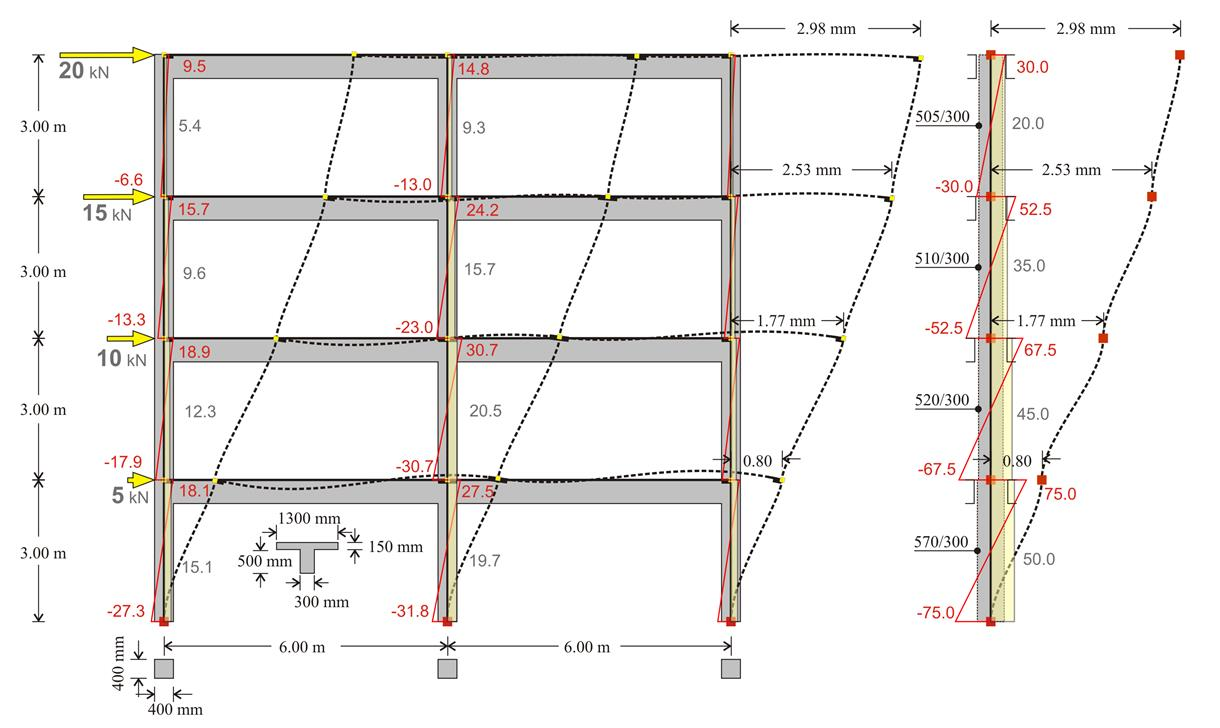Figure 5.3.3-3: FRAME type structure comprising three columns with cross-section 400/400 and equivalent structure of one fixed-ended column per storey
Figure 5.3.3-3: FRAME type structure comprising three columns with cross-section 400/400 and equivalent structure of one fixed-ended column per storey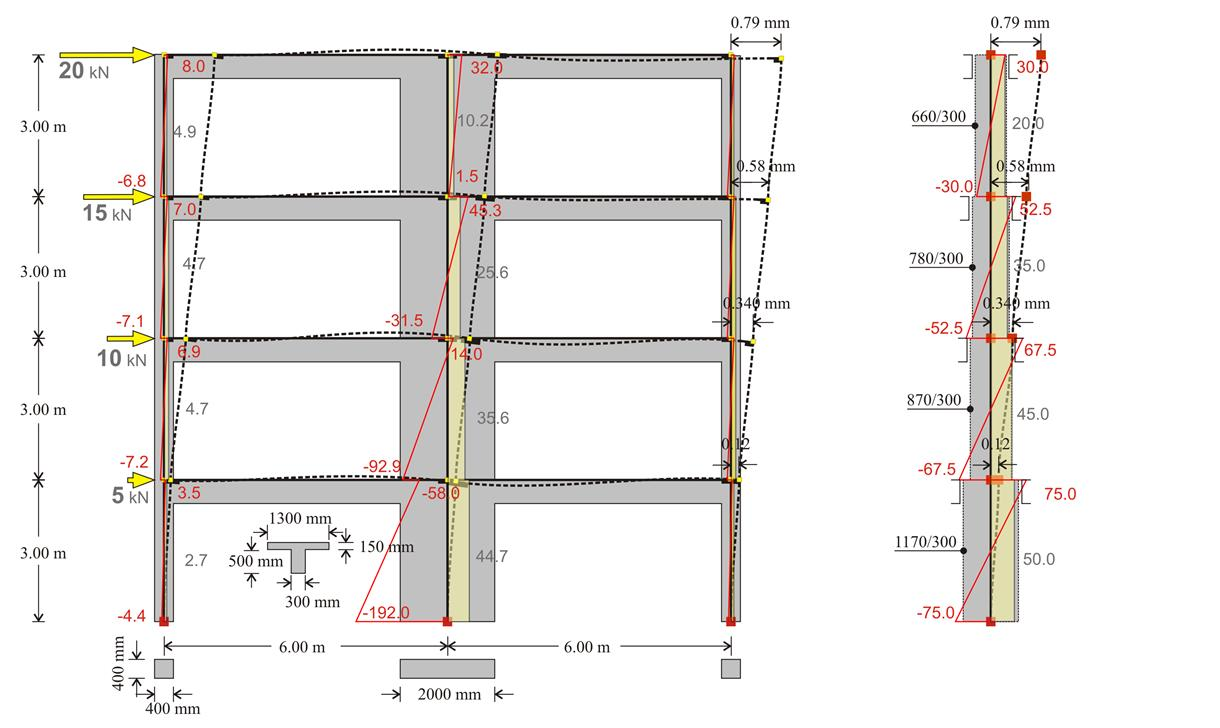Figure 5.3.3-4: DUAL type structure comprising one wall with a cross-section of 2000/300 and two columns a with a cross-section of 400/400 and Equivalent structure of one fixed-ended column per storey
Figure 5.3.3-4: DUAL type structure comprising one wall with a cross-section of 2000/300 and two columns a with a cross-section of 400/400 and Equivalent structure of one fixed-ended column per storey

Note:

The interstorey stiffness variation of the frame type structure is small (equivalent cross-section 570/300 at level 1 and 505/300 at level 4), compared to that corresponding to dual type structure, which is significant (1170/300 and 660/300 respectively).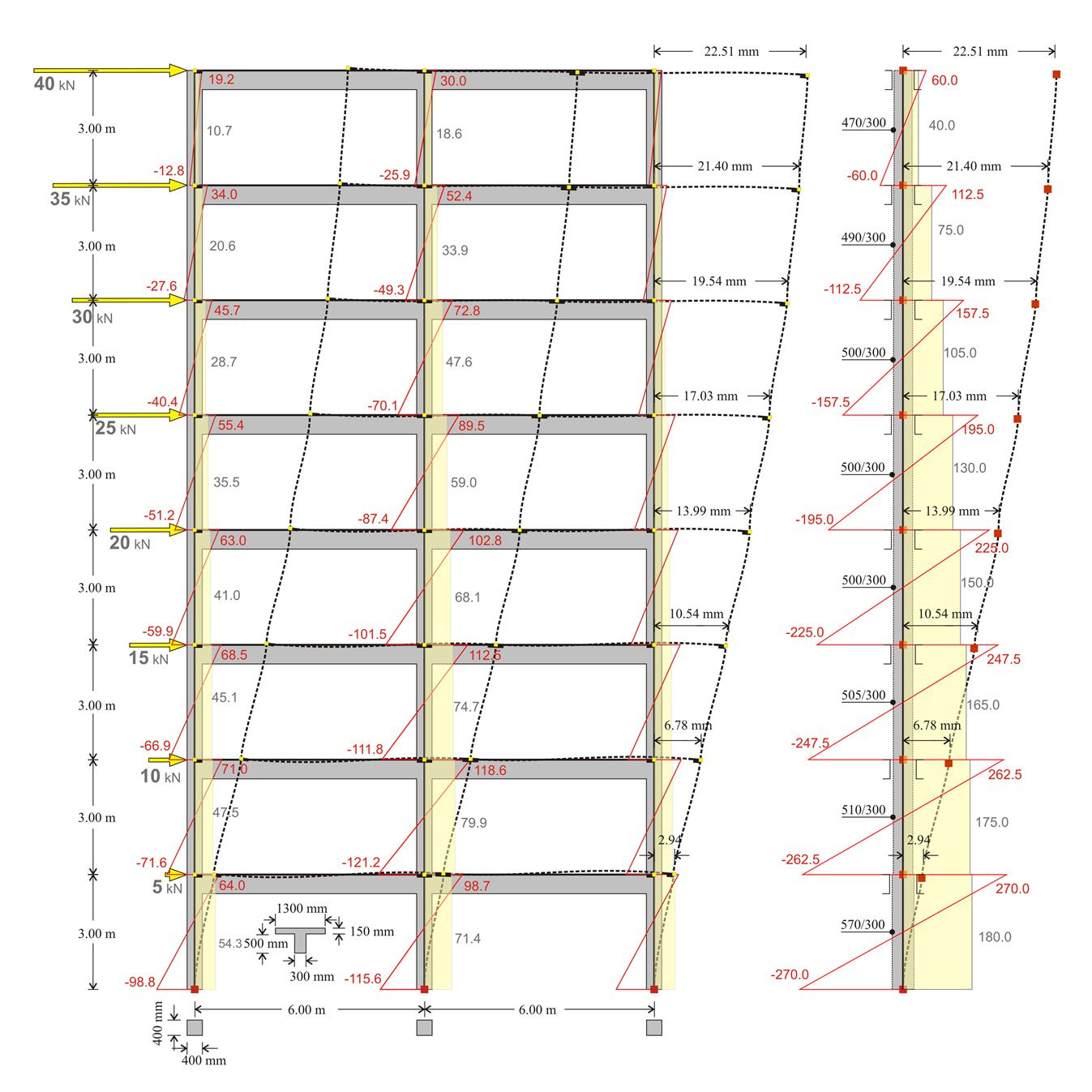Figure 5.3.3-5: FRAME type structure comprising three column with cross-section 400/400 and equivalent structure of one fixed-ended column per storey
Figure 5.3.3-5: FRAME type structure comprising three column with cross-section 400/400 and equivalent structure of one fixed-ended column per storey

Notes:

• In all types of structures, frame or dual, the sum of column shear forces of a storey is equal to the sum of the seismic forces of all the above storeys. Indicatively, for the first storey the sum is 54.3+71.4+54.3=180, while for the last 10.7+18.6+10.7=40. The middle column of the first storey carries the 71.4/180=40% of the total shear force, while each of the end columns carries 30%. In the last storey the middle column carries the 18.6/40=46%, while each of the end columns carries 27%.
• In both frame and dual systems, for each column Mo-Mu=V·h, where Mo is the moment at the top, Mu is the moment at the base, V is the shear force and h is the height of the column. For instance, for the middle column of the previous structure 98.7-(-115.6)=71.4·3.0 (214.3≈214.2).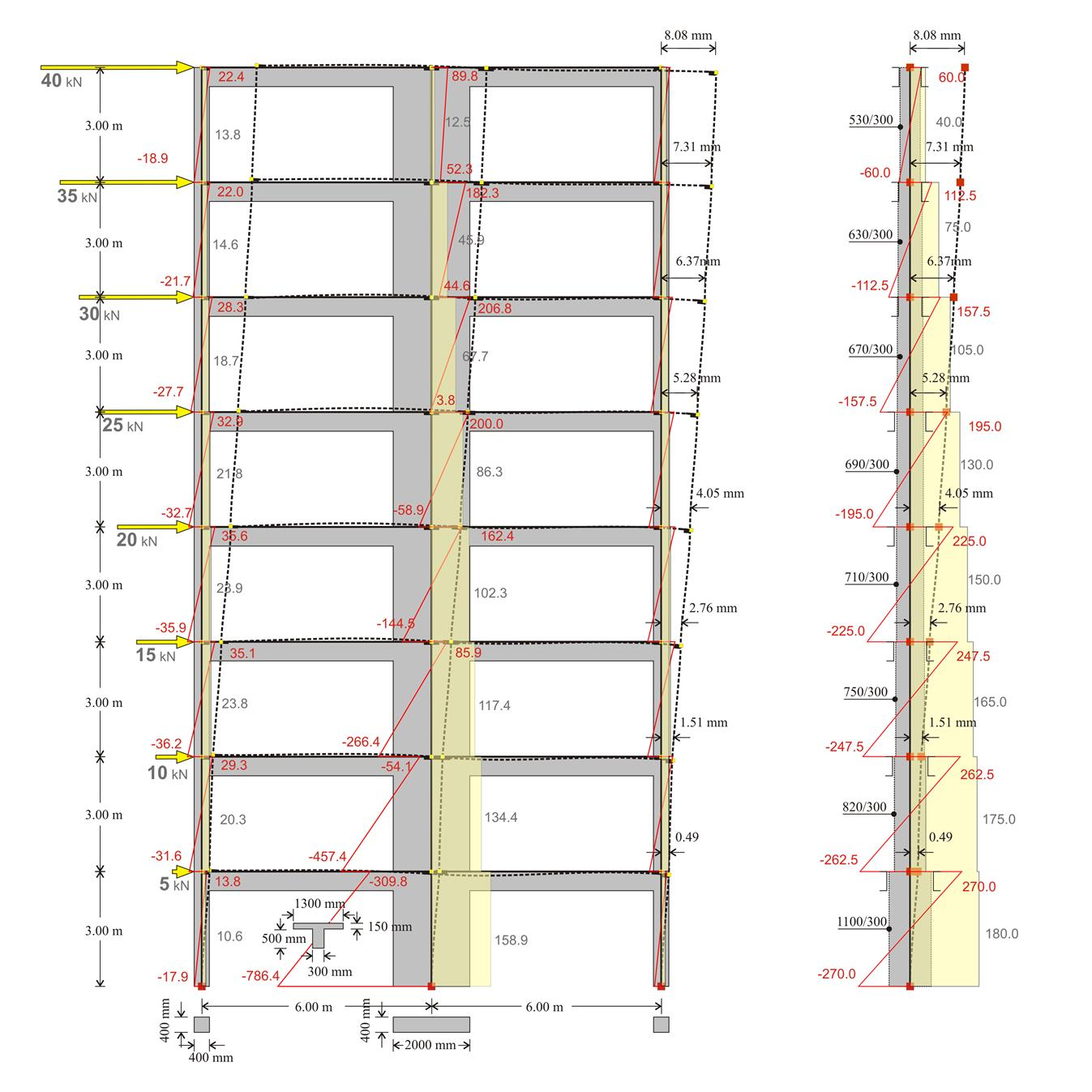Figure 5.3.3-6: DUAL type structure comprising two columns and one wall with cross-sections 400/400 and 2000/300 respectively and equivalent structure of one fixed-ended column per storey
Figure 5.3.3-6: DUAL type structure comprising two columns and one wall with cross-sections 400/400 and 2000/300 respectively and equivalent structure of one fixed-ended column per storey

Notes :

• In the first storey, the sum is 10.6+158.9+10.6=180. The wall carries 158.9/180=88% of the total shear force, while each column carries 11%. In the last storey, the sum is13.8+12.5+13.8=40. The wall carries 12.5/40=32% of the total shear force, while each column 34%. It is concluded that the wall has a favourable effect on the first storey columns, in contrast to that corresponding to the last storey.
• The expression Mo-Mu=V·h, applies for both columns and wall. Indicatively, for the first storey wall -309.9-(-786.4)=158.9·3.0 (476.5≈476.7), while for that of the last storey 89.8-52.3=12.5·3.0 (37.5=37.5).
• The maximum displacement of the dual type structure is equal to 8.08 mm, i.e. almost three times smaller than the one corresponding to the frame type structure (22.51 mm).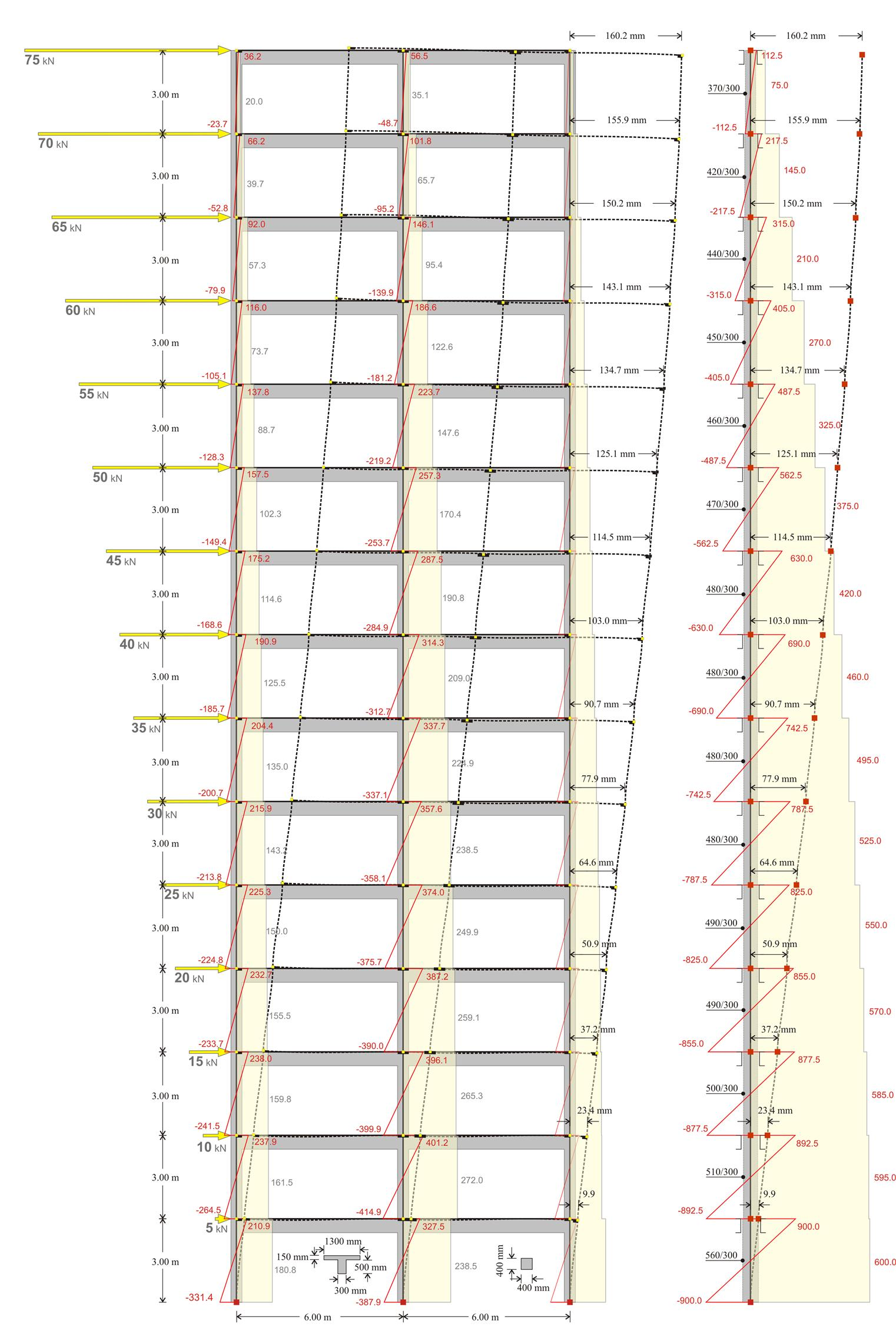Figure 5.3.3-7: FRAME type structure comprising three columns with cross-section 400/400 and equivalent structure of one fixed-ended column per storey
Figure 5.3.3-7: FRAME type structure comprising three columns with cross-section 400/400 and equivalent structure of one fixed-ended column per storey

Note:

It should be emphasised that in all previous examples, the comparison of the two structural systems is important rather than the absolute quantities, which after all derive from specific values of the seismic forces. These values have been selected arbitrarily, yet satisfying the triangular distribution rule.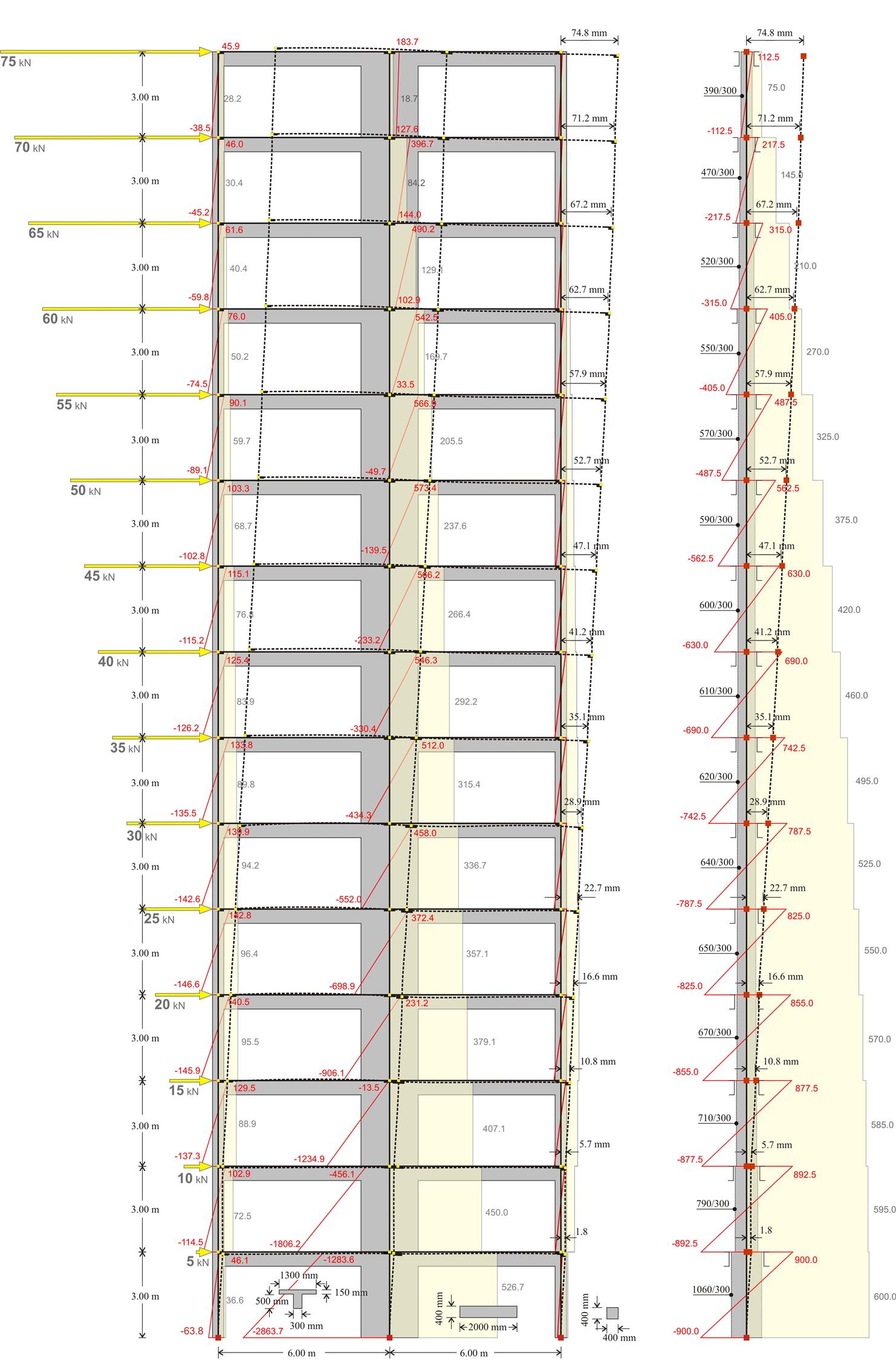Figure 5.3.3-8: DUAL type structure comprising one wall with a cross section of 2000/300 and two columns with a cross section of 400/400 with cross-sections 400/400 and 2000/300 respectively and equivalent structure of one fixed-ended column per storey
Figure 5.3.3-8: DUAL type structure comprising one wall with a cross section of 2000/300 and two columns with a cross section of 400/400 with cross-sections 400/400 and 2000/300 respectively and equivalent structure of one fixed-ended column per storey

Note:

The maximum displacement of the frame type structure is equal to 160 mm, i.e. almost twice of that corresponding to the dual type structure (75 mm).

Comparative table of Frame and Dual structural systems

 Effects Structural System Practical conclusions Frame Dual Displacements magnitude large small Need for wide seismic joint between buildings in frame systems. Earthquake resistance of brick walls small large EC8 prohibits in general using brittle walls e.g. brick walls for High Ductility Class (DCH) (see§6.1.5.4). Fluctuation of interstorey relative displacements and stiffnesses small large In dual systems, walls develop a favourable behaviour in lower while frames develop a favourable behaviour in upper storeys. To this end, the coexistence of both frames and walls in a structure is necessary (dual system). Foundation effect small large Walls should be founded on foundation beams of high stiffness. The more efficient foundation for walls way is on the basement walls ensuring fixity at their base. Shear effect small significant The shear effect should be taken into account in the design of dual systems in contrast to the design of frame systems where it can be omitted. Rigid bodies effect small significant The rigid bodies effect should be taken into account in the design of dual systems while in the design of frame systems it can be omitted.

The behaviour of dual systems is more favourable than frame systems under seismic actions.

« Multistorey plane dual systems Space frames »